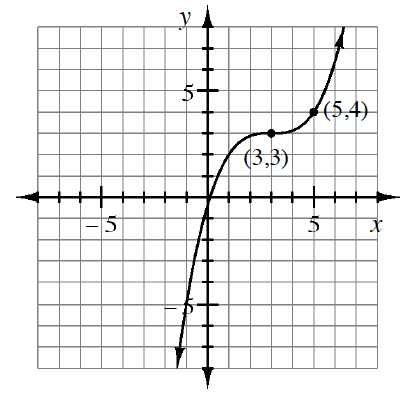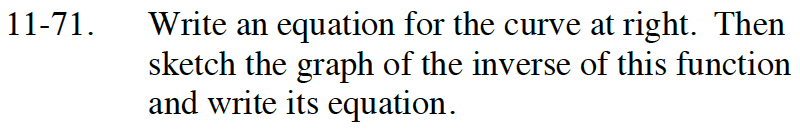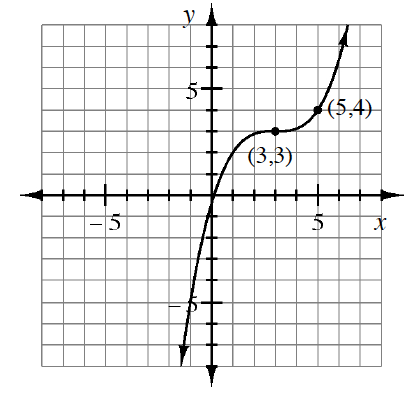Home > INT3 > Chapter 11 > Lesson 11.2.2 > Problem11-71

11-71.
1. Write an equation for the curve below. Then sketch the graph of the inverse of this function and write its equation. Homework Help ✎

2.What parent graph is this similar to?
How is this graph transformed from the parent graph?
Notice that you are given a point other than the locator point so you can solve for a.To find the inverse equation, interchange x and y and solve for y.
To graph the inverse equation, choose some points on this graph and interchange x and y.